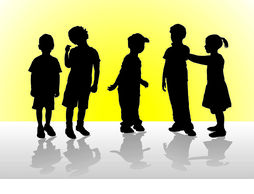# Weight of children riddle

The five school children in couples weigh 129 pounds, 125 pounds, 124 pounds, 123 pounds, 122 pounds, 121 pounds, 120 pound, 118 pounds, 116 pounds and 114 pounds on a weighing machine.

What was the weight of each one of the five children if taken separately?56, 58, 60, 64 and 65 poundswhat logic should we follow  to get the ans ??

on 10th January 2019.I first added all the sums and divided it by 20 giving me an average of about 60 pound. The lowest number is the sum of the two lightest persons. The highest number is the sum of the two heaviest persons. The first try gave me the right weights.

on 11th January 2019.NICE COMMUNITY NICE

on 11th January 2019.That’s pretty smart ..thankyou

on 14th January 2019.

Let the children’s be  A,B,C,D , E
assuming that E > D>C>B>A..
that means,
E+D = maximum weight = 129
E+C = second max = 125
A+B=minimum weight = 114
If you think about it..each person is weighed on the scale EXACTLY 4 times…
i.e            4A+4B+4C+4D+4E = Total weight = 1212 pounds
A+B+C+D+E = 303 pounds
(E+d)+(A+B)+C = 303
C = 60

and you find others weight accordingly….